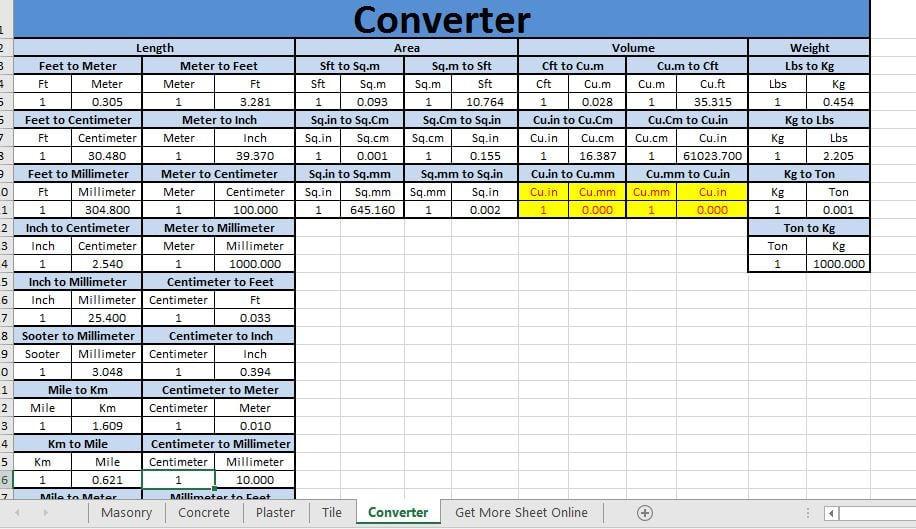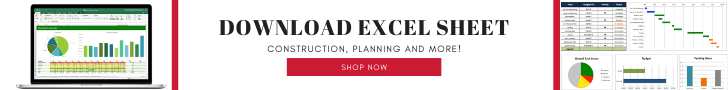Welcome back in World’s No.1 Civil Engineering Blog Expertcivil.com so finally today I’m going to share for “Download Excel Sheet for Civil Work Quantities” Let’s go and Download the spreadsheet or Excel File.

### Why Excel sheet for Civil Work Quantities?

Nice Question, in this sheet all formula is arranged which is very important for calculation so simply you need to put your value and get results directly.### Advantages of Excel Sheet for Civil Work Quantities

Some following advantages are mentioned below:-

1. You can calculate Brick Masonry Work.
• Masonry Volume
• No of Bricks
• Ratio
• Cement
• Sand

2. You can also calculate Concrete Work

• Concrete Work for Ft
• Concrete Work for Meter
• Steel in R.C.C for Meter
• Steel in R.C.C for Ft

3. Also, calculate Plaster Work and Quantities like

• Plaster Work for Ft
• Plaster Work for Meter

4. By using this sheet we can also calculate no of the tiles floor area of tiles

• Tile Work for Ft
• Tile Work for Meter

5. The converter is also attached in the excel sheet so you can also convert any values from mks to cgs or mks to fps whatever. see attached image.### Contents of the Excel Sheet

Here is available all types of formula which are generally used on construction site by the way I’m giving short points of contents of excel sheet.

• Brick Masonry Work
• Block Masonry Work
• Concrete Work
• Plaster Work
• Tile Work
• Converter
• Meter to feet
• Feet to Meter
• Area
• Sft to Sq.m
• Sq.m to Sft
• Feet to Centimeter
• Inch to Centimeter
• Inch to Millimeter
• Sooter to Millimeter
• Mile to Km
• Km to Mile
• Mile to Meter
• Mile to Feet
• Km to Meter
• Km to Feet
• Sq.in to Sq.mm
• Ton to Kg
• Kg to Ton
• Kg to Lbs### This Post Has 2 Comments

1.how we can calculate /m2(Blockwork, Including Mortar thickness)

1.It could be much better if you mention grade of Mortar, work volume of brick masonry and class of bricks too.

Assuming

Grade of mortar = 1:6 (cement : sand)
Class A brick (19 cm x 9 cm x 9 cm)
Volume of brickwork = 1.0 m^3
Thickness of mortar = 10 mm
Step 1 :- Calculation of bricks

No. of bricks = (volume of brick work / volume of one brick with mortar)

Volume of one brick (without mortar) = .19*.09*.09 = 0.001539 m^3

since thickness of mortar = 10 mm (0.01 m)

Volume of brick with mortar = (0.19+0.01) x (0.09+0.1)x (0.09+0.1) = 0.2×0.1×0.1 = 0.002 m^3

therefore,

No.of bricks = 1.0/ (0.002) = 500

consider the percentage of waste as 10 % or 15 %

Total no. of bricks = 500 + (10 x 500 )/100=550

Step 2 :- Calculation for quantity of mortar

Since we need 500 no of bricks

volume occupied by bricks = No of bricks x volume of one brick

Volume of bricks = 500 x 0.001539 = 0.7695 m^3

Volume of mortar = Volume of BRICK WORK – Volume of BRICKS

therefore,

volume of Mortar = 1.0 – 0.7695 = 0.2305 m^3

Step 3:- Calculation for Quantity of CEMENT

Cement = (dry volume of mortar x Cement ratio)/ sum of the ratio,s(proportion)

Dry volume of Mortar = 1.33 x 0.2305 = 0.3065 m^3 ( 33% increment due to volume shrink after water )

cement= (0.3065 x 1) / (1+6) = 0.3065/7= 0.043795 m^3

cement (kg) = 0.043795 x 1440 = 63.0648 kg

No. of bags = 63.0648 / 50 = 1.26 bags approximately 2 bags

Step 4 :- Calculation for Quantity of SAND

Sand = (dry volume of mortar x Sand ratio)/ sum of the ratio,s(proportion)

or

sand = cement volume x 6 ( since 1:6 grade of mortar)

therefore,

sand = 0.043795 = 0.26277 m^3 or

sand = 0.26277 x 1920 = 504.5184 kg /1000 = 0.5045 tonnes or

sand = 0.26277 x 35.3147 = 9.279 cft or

sand = 0.26277 x 0.354 = 0.0930 brass

So, For

Grade of mortar = 1:6 (cement : sand)
Class A brick (19 cm x 9 cm x 9 cm)
Volume of brickwork = 1.0 m^3
Thickness of mortar = 10 mm
Number of Bricks = 500

Cement = 1.26 bags of 50 Kg

Sand = 0.26277 m^3

This site uses Akismet to reduce spam. Learn how your comment data is processed.